The Ideal Gas Constant

Professor John M. Cimbala

Students are often confused by the units of the ideal gas constant. This confusion is compounded by the fact that there are two forms of the gas constant: the universal gas constant and the specific gas constant. To avoid confusion and error, these are defined below, along with their relationships with mol and molecular weight. For completeness, numerical values are given in both S.I. and English units.

Mol, Atomic weight, and Molecular weight

• A mol (sometimes gmol, g-mol, or mole, not to be confused with the rodent) denotes an amount of matter. Specifically one mol is 6.0251 x 1023 molecules of a substance, a standard number of molecules known as Avogadro’s number. Strictly speaking, mol does not have dimensions of mass; rather, mol is a primary dimension in and of itself, i.e. the amount of matter. Note that some authors, however, treat mol as a unit of mass. The number of mols of a substance is denoted by the letter n.
• Molecular weight (M) is defined as the number of grams (g) per mol of the molecular form of a substance. Do not confuse this with Atomic wight (Ar), which is the number of grams per mol of an atom of the substance. Atomic weight is obtained from standard periodic charts of the elements. For example, the atomic weight of nitrogen is listed on periodic charts as Ar,nitrogen = 14.0067 g/mol. However, nitrogen in its gaseous or vapor state occurs as a diatomic molecule, N2; thus, Mgaseous nitrogen = 28.0134 g/mol. Since air is made up predominantly of nitrogen gas, the molecular weight of air is very close to that of nitrogen, i.e.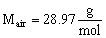• In S.I. units, the kilogram (kg) is preferred over the gram; thus the kilogram-mol (kmol, sometimes kg-mol or kg-mole) is often used instead of the mol. By definition, a kmol is defined as 1000 mol, or 6.0251 x 1026 molecules of the substance. The molecular weight of air in terms of kg and kmol is then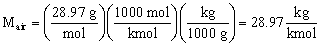• In English units, the pound-mass (lbm) is the standard unit of mass. In order to use the same atomic weights as those listed on the periodic chart, the pound-mol, (lbmol, sometimes lb-mol, lbm-mol, or lbm-mole) is defined. The atomic weight of elemental nitrogen, for example, in English units is Ar,nitrogen = 14.0067 lbm/lbmol, and the molecular weight of air is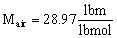Universal gas constant and ideal gas law

• The universal gas constant (Ru) is, as its name implies, universal, i.e. the same regardless of the gas being considered.
• The ideal gas law in terms of Ru is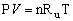where P is the absolute pressure of the gas, V is the volume occupied by the gas, n is the number of mols of the gas, and T is the absolute temperature of the gas.
• In S.I. units,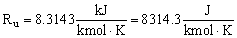In the above form of the ideal gas law, since Ru is given in terms of kmol, n must represent the number of kmols of the substance. The mass, m, of the substance in kg is equal to n times the molecular weight, i.e. m = nM.
• In English units,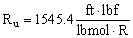In the ideal gas law above, since Ru is given in terms of lbmol, n must represent the number of lbmols of the substance. The mass, m, of the substance in lbm is equal to n times the molecular weight, i.e. m = nM.

Specific gas constant

• The specific gas constant (R, sometimes Rgas) is not universal, and its value depends on the specific gas being considered. R is defined as the universal gas constant divided by the molecular weight of the substance, i.e.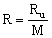The dimensions of R are not the same as those of Ru, since molecular weight is a not a dimensionless quantity, although some authors treat it as such.
• The ideal gas law in terms of R is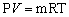where P is the absolute pressure of the gas, V is the volume occupied by the gas, m is the mass of the gas, and T is the absolute temperature of the gas.
• For air in S.I. units,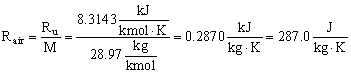• For air in English units,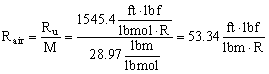• As a check, one can convert from S. I. to English units, i.e.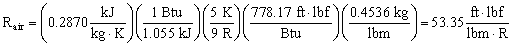The disagreement in the last digit is due to round-off errors in the conversion factors.